Circular Motion and Satellite Motion - Lesson 4 - Planetary and Satellite Motion

# Mathematics of Satellite Motion

The motion of objects is governed by Newton's laws. The same simple laws that govern the motion of objects on earth also extend to the heavens to govern the motion of planets, moons, and other satellites. The mathematics that describes a satellite's motion is the same mathematics presented for circular motion in Lesson 1. In this part of Lesson 4, we will be concerned with the variety of mathematical equations that describe the motion of satellites.

### Orbital Speed Equation

Consider a satellite with mass Msat orbiting a central body with a mass of mass MCentral. The central body could be a planet, the sun or some other large mass capable of causing sufficient acceleration on a less massive nearby object. If the satellite moves in circular motion, then the net centripetal force acting upon this orbiting satellite is given by the relationship

Fnet = ( Msat • v2 ) / R

This net centripetal force is the result of the gravitational force that attracts the satellite towards the central body and can be represented as

Fgrav = ( G • Msat • MCentral ) / R2

Since Fgrav = Fnet, the above expressions for centripetal force and gravitational force can be set equal to each other. Thus,

(Msat • v2) / R = (G • Msat • MCentral ) / R2

Observe that the mass of the satellite is present on both sides of the equation; thus it can be canceled by dividing through by Msat. Then both sides of the equation can be multiplied by R, leaving the following equation.

v2 = (G • MCentral ) / R

Taking the square root of each side, leaves the following equation for the velocity of a satellite moving about a central body in circular motion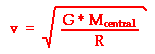where G is 6.673 x 10-11 N•m2/kg2, Mcentral is the mass of the central body about which the satellite orbits, and R is the radius of orbit for the satellite.

### The Acceleration Equation

Similar reasoning can be used to determine an equation for the acceleration of our satellite that is expressed in terms of masses and radius of orbit. The acceleration value of a satellite is equal to the acceleration of gravity of the satellite at whatever location that it is orbiting. In Lesson 3, the equation for the acceleration of gravity was given as

g = (G • Mcentral)/R2

Thus, the acceleration of a satellite in circular motion about some central body is given by the following equation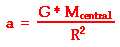where G is 6.673 x 10-11 N•m2/kg2, Mcentral is the mass of the central body about which the satellite orbits, and R is the average radius of orbit for the satellite.

### Orbital Period Equation

The final equation that is useful in describing the motion of satellites is Newton's form of Kepler's third law. Since the logic behind the development of the equation has been presented elsewhere, only the equation will be presented here. The period of a satellite (T) and the mean distance from the central body (R) are related by the following equation: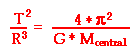where T is the period of the satellite, R is the average radius of orbit for the satellite (distance from center of central planet), and G is 6.673 x 10-11 N•m2/kg2.

There is an important concept evident in all three of these equations - the period, speed and the acceleration of an orbiting satellite are not dependent upon the mass of the satellite.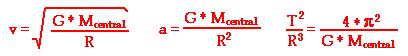None of these three equations has the variable Msatellite in them. The period, speed and acceleration of a satellite are only dependent upon the radius of orbit and the mass of the central body that the satellite is orbiting. Just as in the case of the motion of projectiles on earth, the mass of the projectile has no effect upon the acceleration towards the earth and the speed at any instant. When air resistance is negligible and only gravity is present, the mass of the moving object becomes a non-factor. Such is the case of orbiting satellites.

### Example Problems

To illustrate the usefulness of the above equations, consider the following practice problems.

 Practice Problem #1 A satellite wishes to orbit the earth at a height of 100 km (approximately 60 miles) above the surface of the earth. Determine the speed, acceleration and orbital period of the satellite. (Given: Mearth = 5.98 x 1024 kg, Rearth = 6.37 x 106 m)

Like most problems in physics, this problem begins by identifying known and unknown information and selecting the appropriate equation capable of solving for the unknown. For this problem, the knowns and unknowns are listed below.

 Given/Known: R = Rearth + height = 6.47 x 106 m Mearth = 5.98x1024 kg G = 6.673 x 10-11 N m2/kg2 Unknown: v = ??? a = ??? T = ???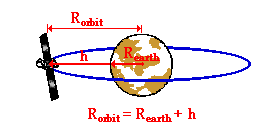Note that the radius of a satellite's orbit can be found from the knowledge of the earth's radius and the height of the satellite above the earth. As shown in the diagram at the right, the radius of orbit for a satellite is equal to the sum of the earth's radius and the height above the earth. These two quantities can be added to yield the orbital radius. In this problem, the 100 km must first be converted to 100 000 m before being added to the radius of the earth. The equations needed to determine the unknown are listed above. We will begin by determining the orbital speed of the satellite using the following equation:

v = SQRT [ (G•MCentral ) / R ]

The substitution and solution are as follows:

v = SQRT [ (6.673 x 10-11 N m2/kg2) • (5.98 x 1024 kg) / (6.47 x 106 m) ]

v = 7.85 x 103 m/s

The acceleration can be found from either one of the following equations:

 (1) a = (G • Mcentral)/R2 (2) a = v2/R

Equation (1) was derived above. Equation (2) is a general equation for circular motion. Either equation can be used to calculate the acceleration. The use of equation (1) will be demonstrated here.

a = (G •Mcentral)/R2

a = (6.673 x 10-11 N m2/kg2) • (5.98 x 1024 kg) / (6.47 x 106 m)2

a = 9.53 m/s2

Observe that this acceleration is slightly less than the 9.8 m/s2 value expected on earth's surface. As discussed in Lesson 3, the increased distance from the center of the earth lowers the value of g.

Finally, the period can be calculated using the following equation:The equation can be rearranged to the following form

T = SQRT [(4 • pi2 • R3) / (G*Mcentral)]

The substitution and solution are as follows:

T = SQRT [(4 • (3.1415)2 • (6.47 x 106 m)3) / (6.673 x 10-11 N m2/kg2) • (5.98x1024 kg) ]

T = 5176 s = 1.44 hrs

 Practice Problem #2 The period of the moon is approximately 27.2 days (2.35 x 106 s). Determine the radius of the moon's orbit and the orbital speed of the moon. (Given: Mearth = 5.98 x 1024 kg, Rearth = 6.37 x 106 m)

Like Practice Problem #2, this problem begins by identifying known and unknown values. These are shown below.

 Given/Known: T = 2.35 x 106 s Mearth = 5.98 x 1024 kg G = 6.673 x 10-11 N m2/kg2 Unknown: R = ??? v = ???

The radius of orbit can be calculated using the following equation:The equation can be rearranged to the following form

R3 = [ (T2 G • Mcentral) / (4 pi2) ]

The substitution and solution are as follows:

R3 = [ ((2.35x106 s)2 (6.673 x 10-11 N m2/kg2) • (5.98x1024 kg) ) / (4 (3.1415)2) ]

R3 = 5.58 x 1025 m3

By taking the cube root of 5.58 x 1025 m3, the radius can be determined as follows:

R = 3.82 x 108 m

The orbital speed of the satellite can be computed from either of the following equations:

 (1) v = SQRT [ (G • MCentral ) / R ] (2) v = (2 • pi • R)/T

Equation (1) was derived above. Equation (2) is a general equation for circular motion. Either equation can be used to calculate the orbital speed; the use of equation (1) will be demonstrated here. The substitution of values into this equation and solution are as follows:

v = SQRT [ (6.673 x 10-11 N m2/kg2)*(5.98x1024 kg) / (3.82 x 108 m) ]

v = 1.02 x 103 m/s

 Practice Problem #3 A geosynchronous satellite is a satellite that orbits the earth with an orbital period of 24 hours, thus matching the period of the earth's rotational motion. A special class of geosynchronous satellites is a geostationary satellite. A geostationary satellite orbits the earth in 24 hours along an orbital path that is parallel to an imaginary plane drawn through the Earth's equator. Such a satellite appears permanently fixed above the same location on the Earth. If a geostationary satellite wishes to orbit the earth in 24 hours (86400 s), then how high above the earth's surface must it be located? (Given: Mearth = 5.98x1024 kg, Rearth = 6.37 x 106 m)

Just as in the previous problem, the solution begins by the identification of the known and unknown values. This is shown below.

 Given/Known: T = 86400 s Mearth = 5.98x1024 kg Rearth = 6.37 x 106 m G = 6.673 x 10-11 N m2/kg2 Unknown: h = ???

The unknown in this problemis the height (h) of the satellite above the surface of the earth. Yet there is no equation with the variable h. The solution then involves first finding the radius of orbit and using this R value and the R of the earth to find the height of the satellite above the earth. As shown in the diagram at the right, the radius of orbit for a satellite is equal to the sum of the earth's radius and the height above the earth. The radius of orbit can be found using the following equation:The equation can be rearranged to the following form

R3 = [ (T2 * G * Mcentral) / (4*pi2) ]

The substitution and solution are as follows:

R3 = [ ((86400 s)2(6.673 x 10-11 N m2/kg2) • (5.98x1024 kg) ) / (4 • (3.1415)2) ]

R3 = 7.54 x 1022 m3

By taking the cube root of 7.54 x 1022 m3, the radius can be determined to be

R = 4.23 x 107 m

The radius of orbit indicates the distance that the satellite is from the center of the earth. Now that the radius of orbit has been found, the height above the earth can be calculated. Since the earth's surface is 6.37 x 106 m from its center (that's the radius of the earth), the satellite must be a height of

4.23 x 107 m - 6.37 x 106 m = 3.59 x 107 m

above the surface of the earth. So the height of the satellite is 3.59 x 107 m.

### Investigate!

There are hundreds of artificial satellites orbiting the Earth. A list of geostationary satellites can be found at http://www.satsig.net/sslist.htm. Use the Satellite Information widget below to explore the various properties - speed, height, orbital path, etc. - of any existing satellite. Simply type in the name (correctly) of the satellite and click on the Get Information button.

1. A satellite is orbiting the earth. Which of the following variables will affect the speed of the satellite?

a. mass of the satellite

b. height above the earth's surface

c. mass of the earth

2. Use the information below and the relationship above to calculate the T2/R3 ratio for the planets about the Sun, the moon about the Earth, and the moons of Saturn about the planet Saturn. The value of G is 6.673 x 10-11 N•m2/kg2.

 Sun M = 2.0 x 1030 kg Earth M = 6.0 x 1024 kg Saturn M = 5.7 x 1026 kg
a. T2/R3 for planets about sun

b. T2/R3 for the moon about Earth

c. T2/R3 for moons about Saturn

3. One of Saturn's moons is named Mimas. The mean orbital distance of Mimas is 1.87 x 108 m. The mean orbital period of Mimas is approximately 23 hours (8.28x104 s). Use this information to estimate a mass for the planet Saturn.

4. Consider a satellite which is in a low orbit about the Earth at an altitude of 220 km above Earth's surface. Determine the orbital speed of this satellite. Use the information given below.

 G = 6.673 x 10-11 Nm2/kg2 Mearth = 5.98 x 1024 kg Rearth = 6.37 x 106 m

5. Suppose the Space Shuttle is in orbit about the earth at 400 km above its surface. Use the information given in the previous question to determine the orbital speed and the orbital period of the Space Shuttle.

Next Section: